##### Statistics: 1,001 Practice Problems For DummiesThe practice problems here will help you understand the standard normal (Z-) distribution, its properties, and how its values are interpreted and used. In the problems below, the random variable X has a normal distribution, with a mean of 17 and a standard deviation of 3.5.

## Sample questions

1. What is the z-score for a value of 21.2?

To calculate the z-score for a value of X, subtract the population mean from x and then divide by the standard deviation: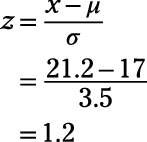2. What is the z-score for a value of 13.5?

To calculate the z-score for a value of X, subtract the population mean from x and then divide by the standard deviation: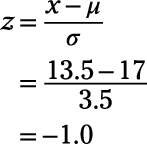3. What value of X corresponds to a z-score of –0.4?

The question gives you a z-score and asks for its corresponding x-value. The z-formula contains both x and z, so as long as you know one of them you can always find the other: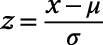You know that z = –0.4,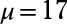and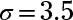so you just plug these numbers into the z-formula and then solve for x: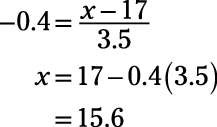4. What value of X corresponds to a z-score of 2.2?

The question gives you a z-score and asks for its corresponding x-value. The z-formula contains both x and z, so as long as you know one of them you can always find the other:You know that z = 2.2,andso you just plug these numbers into the z-formula and then solve for x: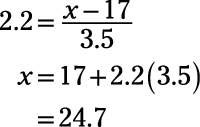If you need more practice on this and other topics from your statistics course, visit 1,001 Statistics Practice Problems For Dummies to purchase online access to 1,001 statistics practice problems! We can help you track your performance, see where you need to study, and create customized problem sets to master your stats skills.